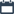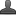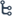## Friday, October 23, 2020

•October 23, 2020
••, ,
•No comments

## Important questions for 10th class Maths chapter-1 Real numbers,Mathematics questionnaire-xclass

MATHEMATICS QUESTIONNAIRE – X CLASS

1. REAL NUMBERS

Hi friends and my dear students! In this post, I have covered Important questions for 10th class maths chapter-1 Real numbers, Chapter wise previous year Questions. After Reading  Please do share it with your friends. Learn Maths for All Classes here.

1 MARK QUESTIONS:

1. State Euclid’s division algorithm

2. Expand (i) log p2q3/r (ii) log15 (iii) logx2y3z4 (iv) log343/125 (v) log 125/625

(vi) logx3/y3(vii) log 64/243

3. Explain why i) 17x11x2+17x11x5

ii) 7x11x13+13 iii)7x6x5x4x3x2x1+5 is a composite number

4. Determine i. Log2 512

ii. log x√ x

iii. log21/16

iv. 22 + log23

v. log20.0001

vi. log10cos0

vii . 22 + log 26

viii log11

ix. 6log67

x. 52 + log 510

xi. 2log3+3log2+log5-log12

xii log10 3+ log10 4+ log10 4- log10 6

xiii. log1218+log128

xiv. log(a2xb3)-log(a3/b2)

xv. logba.logcb.logac2/3

5. Write the following in exponential form

i. log10 32= x

ii. log5 625=  y

iii. log10 1000=  z

iv. log7 1/343= -a

v. log100.001=-4

vi.log5125=3

6. Find the HCF of the smallest composite number and the smallest prime number?

7. If HCF (306,657) = 9,find LCM

8. Write the condition to be satisfied by ‘q’ so that a rational number p/q has a terminating decimal expansion?

2 MARK QUESTIONS:

9. Find the LCM and HCF of (i) 12, 18 (ii) 12,15,21 (iii) 23 x 32 and 24 x 3 by prime factorization method

10. Use Euclid’s algorithm to find the HCF of (i) 900,270 (ii) 96,72

11. Find the HCF and LCM of 75 and 160 by the fundamental theorem of Arithmetic and verify LCM x HCF =product of two numbers

12. Solve i. 3x=5x-2

ii. 2x+1=31-x

iii. 3x=5x+2

13. Show that

i. 7 √5

ii. 3√2

iii. 3+2√5

iv. 5-√3

v. 1/√2

vi. √26 is irrational

14. Write i. 2log3+3log5-5log

ii. log 10+2log3-log2

iii. 2logx+3log4+log2

iv. 2log3-1/2log16+log12

as a single logarithm

15. Show that i. 4n ii. 6n iii.12n,n€N can never end with the digit 0

16. State whether the following are terminating or non- terminating repeating decimal without actual division

i.29/343 ii.23/2252 iii.6/15 iv.35/50 v.7/8 vi.13/3125 vii. 9/15  viii. 77/210

17. Find the value of a+b+c+d if product of first ten natural numbers is written as 2ax3bx5cx7d

18. If the prime factorization of natural number (n) is 23x32x52x7. How many consecutive zeroes will it have at the end of it justify your answer?

19. If log2(x2-4x+7)=2 find the value of ’x’

Also Check

Introduction To Trigonometry

Introduction to Knowing Our Numbers Key Points

SSC (10th class) Trigonometry Exercise - 11.1 Solution

SSC(10th class) Trigonometry Exercise - 11.1 Solutions

4 MARK QUESTIONS:

1. Use Euclid’s division lemma to show that any positive odd integer is of the form 6q+1 or 6q+3 or 6q+5 where q is integer

2. Use Euclid’s lemma to show that square of any positive integer is of form 3p,3p+1

3. Show that i.

are irrationals.

4. If log(x+y/3) =1/2(logx+logy) then find the value of x/y+y/x.

5. If i.x2+y2=25xy then show that 2log(x+y)=3log3+logx+logy ii.x2+y2=6xy then show that 2log(x+y)=3log2+logx+logy

6. (2.3)x=(0.23)y = 1000 then find the value of 1/x-1/y

7. Show that a positive odd integer is of the form 4q+1 or 4q+3 where q is an integer

8. Show that If x2+y2 =3xy then 2log(x-y) = logx + logy ii.x2+y2=10xy, 2log(x+y)=logx+logy+2log2+log3

9. If a2+b2=7ab then log(a+b/3)=1/2(loga+logb)

10. Use Euclid’s division lemma to show that the cube of any positive integer is of the form 9m,9m+1,9m+8

PRIORITY-II

1 MARK QUESTIONS:

1. Sate fundamental theorem of arithmetic

2. If LCM of two numbers ’a’ and ‘b’ is 24 and their HCF is ‘1’ then find the numbers

3. If log10x=a, write 102a-3 value in terms of x

4. Define logarithm of a natural number?

5. Is log110 defined? Why?

6. Write any rational number between 2/3 & 3/5?

7. Find the value of log108 when log2=0.3010

8. Can you find the HCF of 7 and 9 without using prime factorization method? Justify your answer?

9. If x=9, y=log32 then find xy

10. What can you say about the LCM and HCF of any two consecutive numbers and prime numbers?

2 MARK QUESTIONS:

11. Write any four laws of logarithms?

12. Is i. log2  ii. log3  iii. log100  iv ∏ . a rational or irrational? justify your answer

13. Do you think that sum of two irrational and product of two irrational is again an irrational? Justify your answer

14. Find ‘x’ if i.2 log+1/2 log9-log3=logx

ii. logx10 = -3

iii. log10x-9 log10x=1

15. Why 6n+5n always end with 1? Explain

16. Prove that logaa=1

17. What is the difference between rational and irrational numbers expressed in decimal form?

18. Show that log27540=2log2+4log3+log5+log17

19. Insert 4 rational numbers between ¾ and 1 without using the a+b/2 formula

20. A number when divided by 61 gives 21 as quotient and 32 as remainder find the number

21. Check whether (2√3+√5) (2√3-√5 is rational or irrational? Justify your answer

4 MARK QUESTIONS:

1. Show that one and one out of n,n+2, or n+4 is divisible by 3 where ‘n’ is any positive integer

2. Show that every positive even integer is of the form 2q and that every positive odd integer is of the form 2q+1 where ‘q’ is some integer

3. Plot√2,√3,√5on number line

4. Prove that logaxy=logax+logay

5. Use Euclid’s division lemma to show that the cube of any positive integer is of the form 7m or 7m+1 or 7m+6

1 MARK QUESTIONS:

1. State Euclid’s division algorithm

2. Expand (i) log p2q3/r (ii) log15 (iii) logx2y3z4 (iv) log343/125 (v) log 125/625(vi) log√  x3/y3(vii) log 64/243

3. Explain why i) 17x11x2+17x11x5

ii) 7x11x13+13 iii)7x6x5x4x3x2x1+5 is a composite number

4. Determine i. Log2 512

ii. log x√ x

iii. log21/16

iv. 22 + log23

v. log20.0001

vi. log10cos0

vii . 22 + log 26

viii log1 1

ix. 6log67

x. 52 + log 510

xi. 2log3+3log2+log5-log12

xii log10 3+ log10 4+ log10 4- log10 6

xiii. log1218+log128

xiv. log(a2xb3)-log(a3/b2)

xv. logba.logcb.logac2/3

5. Write the following in exponential form

i. log10 32= x

ii. log5 625=  y

iii. log10 1000=  z

iv. log7 1/343= -a

v. log100.001=-4

vi.log5125=3

6. Find the HCF of the smallest composite number and the smallest prime number?

7. If HCF (306,657) = 9,find LCM

8. Write the condition to be satisfied by ‘q’ so that a rational number p/q has a terminating decimal expansion?

## Trigonometry Do This & Try this solution

2 MARK QUESTIONS:

9. Find the LCM and HCF of (i) 12, 18 (ii) 12,15,21 (iii) 23 x 32 and 24 x 3 by prime factorization method

10. Use Euclid’s algorithm to find the HCF of (i) 900,270 (ii) 96,72

11. Find the HCF and LCM of 75 and 160 by fundamental theorem of Arithmetic and verify LCM x HCF =product of two numbers

12. Solve i. 3x=5x-2

ii. 2x+1=31-x

iii. 3x=5x+2

13. Show that i. 7 √5

ii. 3√2

iii. 3+2√5

iv. 5-√3

v. 1/√2

vi. √26 is irrational

14. Write i. 2log3+3log5-5log

ii. log 10+2log3-log2

iii. 2logx+3log4+log2

iv. 2log3-1/2log16+log12

as a single logarithm

15. Show that i. 4n ii. 6n iii.12n,nN can never end with the digit 0

16. State whether the following are terminating or non- terminating repeating decimal without actual division

i.29/343 ii.23/2252 iii.6/15 iv.35/50 v.7/8 vi.13/3125 vii. 9/15  viii. 77/210

17. Find the value of a+b+c+d if the product of the first ten natural numbers is written as 2ax3bx5cx7d

18. If the prime factorization of natural number (n) is 23x32x52x7. How many consecutive zeroes will it have at the end of it justify your answer?

19. If log2(x2-4x+7)=2 find the value of ’x’

4 MARK QUESTIONS:

1. Use Euclid’s division lemma to show that any positive odd integer is of the form 6q+1 or 6q+3 or 6q+5 where q is integer

2. Use Euclid’s lemma to show that square of any positive integer is of form 3p,3p+1

3. Show that i.

are irrationals.

4. If log(x+y/3) =1/2(logx+logy) then find the value of x/y+y/x.

5. If i.x2+y2=25xy then show that 2log(x+y)=3log3+logx+logy ii.x2+y2=6xy then show that 2log(x+y)=3log2+logx+logy

6. (2.3)x=(0.23)y = 1000 then find the value of 1/x-1/y

7. Show that a positive odd integer is of the form 4q+1 or 4q+3 where q is integer

8. Show that If x2+y2 =3xy then 2log(x-y) = logx + logy ii.x2+y2=10xy, 2log(x+y)=logx+logy+2log2+log3

9. If a2+b2=7ab then log(a+b/3)=1/2(loga+logb)

10. Use Euclid’s division lemma to show that the cube of any positive integer is of the form 9m,9m+1,9m+8

PRIORITY-II

1 MARK QUESTIONS:

1. Sate fundamental theorem of arithmetic

2. If LCM of two numbers ’a’ and ‘b’ is 24 and their HCF is ‘1’ then find the numbers

3. If log10x=a, write 102a-3 value in terms of x

4. Define logarithm of a natural number?

5. Is log110 defined? Why?

6. Write any rational number between 2/3 & 3/5?

7. Find the value of log108 when log2=0.3010

8. Can you find the HCF of 7 and 9 without using prime factorization method? Justify your answer?

9. If x=9, y=log32 then find xy

10. What can you say about the LCM and HCF of any two consecutive numbers and prime numbers?

2 MARK QUESTIONS:

11. Write any four laws of logarithms?

12. Is i. log2 ii. log3 iii. log100 iv ∏ . a rational or irrational? justify your answer

13. Do you think that sum of two irrational and product of two irrational is again an irrational? Justify your answer

14. Find ‘x’ if i.2 log+1/2 log9-log3=logx

ii. logx10 = -3

iii. log10x-9 log10x=1

15. Why 6n+5n always end with 1? Explain

16. Prove that logaa=1

17. What is the difference between rational and irrational number expressed in decimal form?

18. Show that log27540=2log2+4log3+log5+log17

19. Insert 4 rational numbers between ¾ and 1 without using a+b/2 formula

20. A number when divided by 61 gives 21 as quotient and 32 as remainder find the number

21. Check whether (2√3+√5) (2√3-√5 is rational or irrational? Justify your answer

4 MARK QUESTIONS:

1. Show that one and one out of n,n+2 or n+4 is divisible by 3 where ‘n’ is any positive integer

2. Show that every positive even integer is of the form 2q and that every positive odd integer is of the form 2q+1 where ‘q’ is some integer

3. Plot√2,√3,√5on number line

4. Prove that logaxy=logax+logay

5. Use Euclid’s division lemma to show that the cube of any positive integer is of the form 7m or 7m+1 or 7m+6